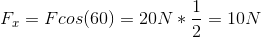# AP Physics C: Mechanics : Interpreting Force Diagrams

## Example Questions

### Example Question #3 : Newton's Laws And Force Diagrams

A 1000kg rocket has an engine capable of producing a force of 30000N. By the third law of motion, when the rocket launches it experiences a reaction force that pushes it upwards of equal magnitude to the force produced by the engine. What is the acceleration of the rocket?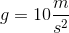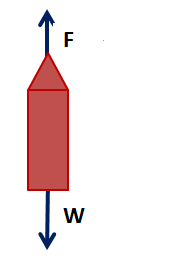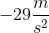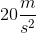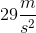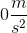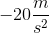Explanation:

When the rocket launches it produces a downward force of 30000N. Due to the third law of motion, the rocket experiences a 30000N reaction force that pushes it upwards.

In addition, the rocket experiences the downward force of its own weight. This is given by: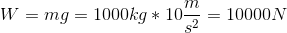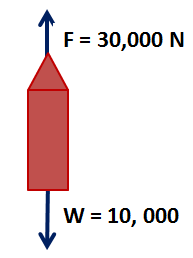We know that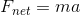.

We know the mass of the rocket is 1000kg, so we need only to find the net force to solve for acceleration.

We know that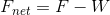(sinceis directed upwards and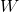is directed downwards).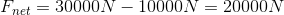Finally we solve for acceleration: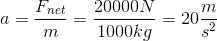### Example Question #1 : Newton's Laws And Force Diagrams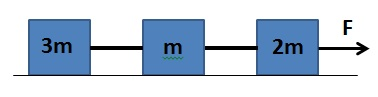Three boxes tied by two ropes move across a frictionless surface pulled by a forceas shown in the diagram. Which of the following is an expression of the acceleration of the system?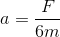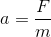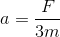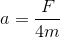None of theseExplanation:

Since the boxes are all connected by ropes, we know that the acceleration of each box is exactly the same. They all move simultaneously, in tandem, with the same velocity and acceleration.

A quick analysis of each box will produce a very simple system of equations. Each rope experiences some tension, so we will label the tension experienced by the rope betweenand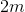as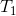. Similarly, we will label the tension experienced by the rope between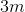andas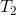.

For the box on the right (of mass) we know that the forcepulls to the right and the rope pulls it to the left with a tension of.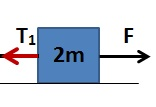We get the equation: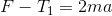is the mass of the box andis its acceleration (which is the same for the other boxes).

For the middle box (of mass) we have a rope pulling on it on the right with tensionand a rope pulling on the left with tension.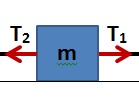So we get the equation: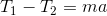is the mass of the box andis its acceleration (which is the same for the other boxes).

Finally, for the box on the left (of mass) we have only one rope pulling it to the right with a tension.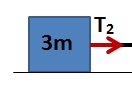So we get the equation: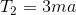is the mass of the box andis its acceleration (which is the same for the other boxes).

Now it is just a matter of simple substitution. We have three equations:From them we can get an expression for the force. Isolatein the second equation.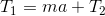Substitute the expression offrom the first equation and simplify.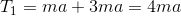Use this value ofin the third equation to get an expression for the force.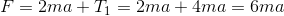So we have: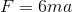Solve for acceleration.### Example Question #5 : Newton's Laws And Force Diagrams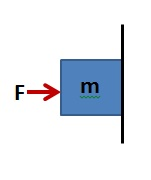A box is being pushed against a wall by a forceas shown in the picture. If the coefficient of static friction between the box and the wall is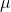, which of the following expressions represents what the force must be for the box not to fall?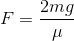None of these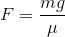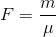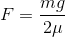Explanation:

This question requires a 2-dimensional analysis. First identify all the forces acting on the box. Since the box is being pushed against a surface it automatically experiences a normal force. The box experiences the downward force of its own weight given by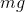. Finally, since the box is trying to slide down, frictionopposes this motion. The following diagram shows these forces: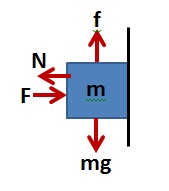The key is that the box should NOT move.

For the horizontal axis, net force must be zero. The two horizontal forces are the applied force and the normal force.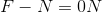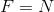Now, on the vertical axis we have fiction and the object's weight: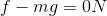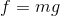Finally, we use the equation for friction force to solve the problem.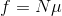Substitute the weight, since it is equal to the force of friction.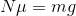Isolate the normal force.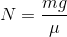Since the normal force is equal to the applied force, this is our final expression.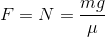### Example Question #6 : Newton's Laws And Force Diagrams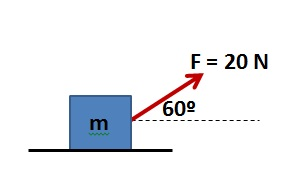A box of mass 10kg is pulled by a force as shown in the diagram. The surface is frictionless. How much force is the box experiencing along the horizontal axis?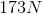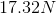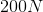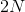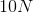Explanation:

You need only to obtain the horizontal component of the force. To do this you must use trigonometric properties.

We see that the force makes an angle of 60º with the horizontal axis. This means that the horizontal component of the force is adjacent to this angle. We can view the diagram as a triangle. From trigonometry we know that to calculate the adjacent side of a triangle we need to multiply the hypotenuse by the cosine of the angle.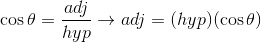We can solve for the horizontal component of the force using the applied force as the hypotenuse: